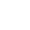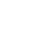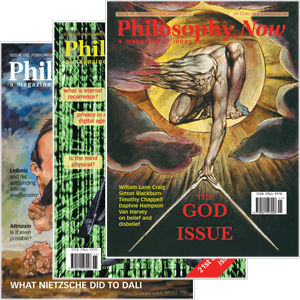# The Limits of Computation

### Apostolos Syropoulos goes back to BASICs to consider whether the human brain is a computer.

There is a school of thought that assumes that all intellectual problems can potentially be solved by computers [for example, see ‘The Future of Philosophy is Cyborg’, by Phil Torres in Issue 141]. However, is this really the case? Here I will try to explain why we do not know where the limits of computability are, and how this lack of knowledge affects our understanding in a number of areas.

Roughly, we can say that a computable (or solvable) problem is any problem that can be solved by a finite set of simple actions. Alternatively, we could say that a computable problem is anything that can be reduced to a mathematical equation and solved accordingly. For example, if someone has to travel to a number of towns and has to schedule her trip so that she has to go through each town only once, this is a mathematical problem. One may say that at first this does not look like a mathematical problem; but we can reduce it to, or transform it into, a mathematical problem (in topology). And if the number of towns that our pilgrim has to travel is small enough, then it is relatively easy to compute an answer. But when the number of towns becomes large enough, then it might not be possible to come up with a road map that goes through each town only once.

Although there are a number of seemingly precise definitions of ‘computability’, it is an imprecise and vague notion. Nevertheless, one can use a Turing machine to rigorously define computation. This (purely conceptual) machine bears the name of the British mathematician Alan Mathison Turing, who defined it in order to solve a famous problem posed by the German mathematician David Hilbert. In a nutshell, Hilbert wanted to make it possible to automatically derive every true mathematical statement. Among other things, this means he was envisioning a machine that could decide whether any stated problem was mathematically calculable, outputting either ‘yes’ or ‘no’ .

It seems that Hilbert’s work in Euclidean geometry was responsible for this idea. Hilbert managed to correct some errors in Euclid’s Elements, and he defined an updated, non-Euclidean form of geometry in his Grundlagen der Geometrie (Foundations of Geometry, 1899). In geometry, there are a number of axioms (undeniable truths or foundational principles) which one uses to prove or disprove any geometrical statement. But Hilbert’s idea was that if this is possible with geometry in particular, it should be possible to prove or disprove any statement in mathematics in general. To realize this possibility, Hilbert tried to make mathematics into a symbol manipulation game. In this game, statements are represented by sequences of otherwise meaningless symbols that are transformed by symbol manipulation rules: for example, the rule ‘aabaa ⟶ cbc’ specifies that the symbol sequence ‘aabaa’ should be replaced by ‘cbc’. After applying some or all of the rules to some axioms – that is, to a starting set of symbol sequences, or strings, as they are more commonly known – one gets a new string. So if the axioms represent the basic truths of Euclidean geometry, and the rules of manipulation are the laws of geometry, then the new string will represent a new true statement in the same geometry. And if you program in other axioms and rules, you might generate all the statements of mathematics in a similar way. Generally speaking, this is Hilbert’s dream.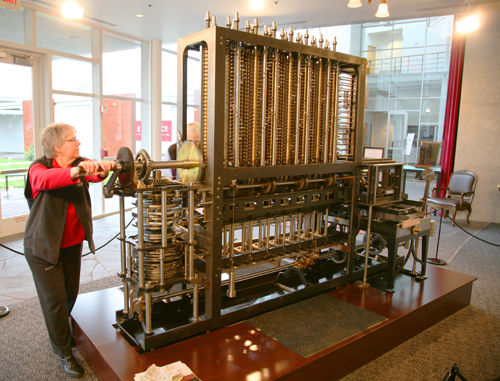Difference Engine, designed by Charles Babbage (1791-1871). Cranking out the solution to a polynomial function.
Taken by Jitze Couperus at the Computer History Museum in Mountain View, CA. Creative Commons 2.0 Licence

## The Limits of Mathematics

In 1931, the Austrian mathematician Kurt Gödel published a paper that showed that Hilbert’s program was impossible. Gödel proved mathematically that there are true mathematical statements that cannot be proved with Hilbert’s game. Gödel’s famous incompleteness theorems thus killed Hilbert’s dream. (His dream is not entirely dead, since there are computer systems used to derive formal proofs, and these theorem provers are quite useful tools.) A few years later, Alonzo Church and his student Turing, working independently, proved that there are formal statements in logic that contain problems that are undecidable. Turing defined a conceptual computing device (that is, a computer that works only in our minds) which can in principle solve any computable problem. Roughly, Turing proved that when this machine stalls, it’s not possible to tell why this happens. When the program stops responding, we cannot tell whether it’s doing something that takes a long time to compute, or whether it’s simply repeating something over and over again because there’s some problem of uncomputability. Telling the difference is called the halting problem. Turing proved that this unsolvability means that there are unsolvable mathematical problems. So this is also fatal to Hilbert’s dream.

At the time that Turing completed this work, there were three theoretical models of computation: Church’s λ-calculus, the Turing machine, and Gödel’s recursive functions. Turing showed that these three formulations of the notion of computation are equivalent: if one can compute something – solve a maths problem – with one of them, it’s also possible to compute it with the other two. This equivalence is behind the Church-Turing thesis, which, roughly, says that if something cannot be computed by a Turing machine (or by the λ-calculus, or by recursive functions), then it cannot be computed. In different words, the Church-Turing thesis sets a limit to what can be computed. The interesting philosophical question is: Is it possible to compute something beyond the reach of these ways of doing mathematics, and if so, how?

In one sense, the Church-Turing thesis is somewhat like Euclid’s Fifth Postulate, which can be paraphrased as: Given a line, and a point not on it, exactly one line parallel to the given line can be drawn through the point. For most people this seems so obvious that no one can refute it. In other words, for most people it seems that there is no way that we can draw more than one line parallel to the given line through the point. The interesting news is that this isn’t true! For instance, if one assumes instead that no lines parallel to the given line can be drawn through the point, then we get what is called elliptic geometry. And if the ‘no lines’ part is replaced by ‘infinitely many lines’, then we get what is called hyperbolic geometry. This was precisely the sort of break from Euclid that Hilbert was interested in.

Transferring the idea from geometry to computing, if we assume that the Turing machine is an archetypal computing device, but now replace it with another computational device that can solve problems the Turing machine cannot solve, then obviously the Church-Turing thesis would be false. But is it possible?

In order to answer this question, we need to understand how the Turing machine operates.

## Touring With Turing

A (hypothetical) Turing machine consists of a tape divided into cells, with a scanning head that stands over a cell, and which can move to the previous or the next cell. It can read what’s on a cell; it can write something on a cell; and it can erase what’s on a cell. In the most simple version of the machine, it is only able to print and read the symbols 1 and 0. If on a cell is a 1 and the scanning head prints a 0, this means that the contents of the cell have been erased. Depending on the machine’s current state – or in other words, depending on the symbol printed on the cell that the head is scanning – the machine may print something on the current cell; move to the previous or to the next cell; or stop. One can construct a Turing machine to solve a particular computable mathematical problem using just these possibilities, similar to using a programming language to construct algorithms to solve a particular problem. Importantly, the machine is assumed to operate in finite time – that is, we cannot wait forever for the machine to complete its operations.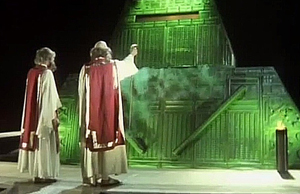Hitchhikers’ Guide to the Galaxy: mega-computer Deep Thought searches for the answer to a philosophical problem.

By extending or modifying the characteristics of the Turing machine, we can produce a new conceptual computing device, with different properties. Jaako Hintikka and Arto Mutanen defined just such an alternative computing device, known as a TAE-machine. A TAE-machine is a Turing machine with an extra tape, which is called the bookkeeping tape. These machines are able to compute things Turing machines fail to compute. In principle, a TAE-machine can be viewed as a counterpart of a quantum computer, which uses quantum phenomena to perform many computations simultaneously. But all these different hypothetical computing machines, and many more, have one thing in common: they cannot solve their own halting problem. However, they are able to solve the halting problems of other machines. This means that we can build a ladder of machines where a given machine can solve the halting problems of all machines that are below it – in other words, it can say with a ‘yes’ or ‘no’ whether the problem which has caused the other machine to halt is computable or not. Our next question is: What machine is on the top of the ladder? This apex machine would be able to decide the limits of computability for all other machines, and so define the absolute limits of com putability.

Very few of these machines have been actually built, so far. For some of them there are no physical obstacles that would prohibit their being constructed, but there are some that seemingly violate the laws of nature, like the infinite time Turing machines proposed by Jeffrey Kidder and Joel David Hamkins, which require infinite time to conclude their operation. (A computer that travels in the space between the outer and the inner event horizon is capable of performing an infinite number of actions in finite time, that is, it is able to perform a supertask. Thus such a machine is a realization of an Infinite time Turing machine. Whether we can actually put a machine in this spacetime, is currently unknown.)

Each machine on the ladder exploits certain physical laws to solve the halting problem of another machine. But of course, our knowledge of the laws of nature is far from complete, so we cannot now say for sure what kind of device would be at the top. Can we even speculate about it? Not really, since the ladder of potentially unsolvable problems is really infinite, but our knowledge of things is quite limited. If and when we will have a comprehensive-enough understanding of nature, then we will be able to go up this ladder, but not now.

In 1969 Konrad Zuse proclaimed that the whole universe is a cellular automaton – a computing device. But if we do not know all the laws of the universe, and, consequently, have no idea what the ultimate computing device is, then how can we say that the universe is a cellular automaton? The same question applies to human thought. Can we now say that the human brain is a computing device? Proponents of mechanism in philosophy assume that the mind is like a machine. Following the American philosopher John Searle, one might say that the mind is a biological machine, but that it does not operate as a symbol manipulation device as a Turing machine does. Searle is famous for his ‘Chinese Room’ argument, which aimed to show that the human mind is more than a symbol manipulator. Roughly, the argument is that someone who does not speak Chinese can perfectly answer questions in Chinese by just manipulating symbols in Chinese (that is, Chinese words) according to a big book of rules. However, there is no understanding of Chinese involved: the operator doesn’t know what the symbols mean. But a mind’s normal operation does involve such understanding of what it’s thinking. Therefore the human mind evidently transcends the computing capabilities of Turing machines.